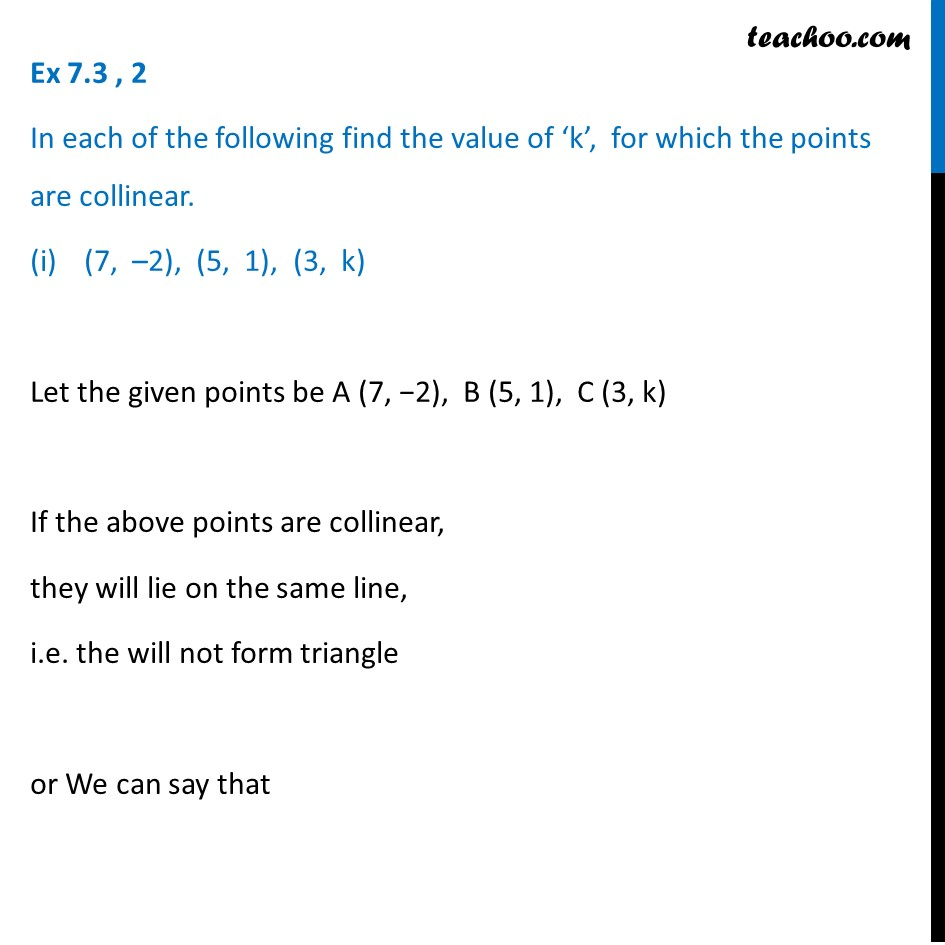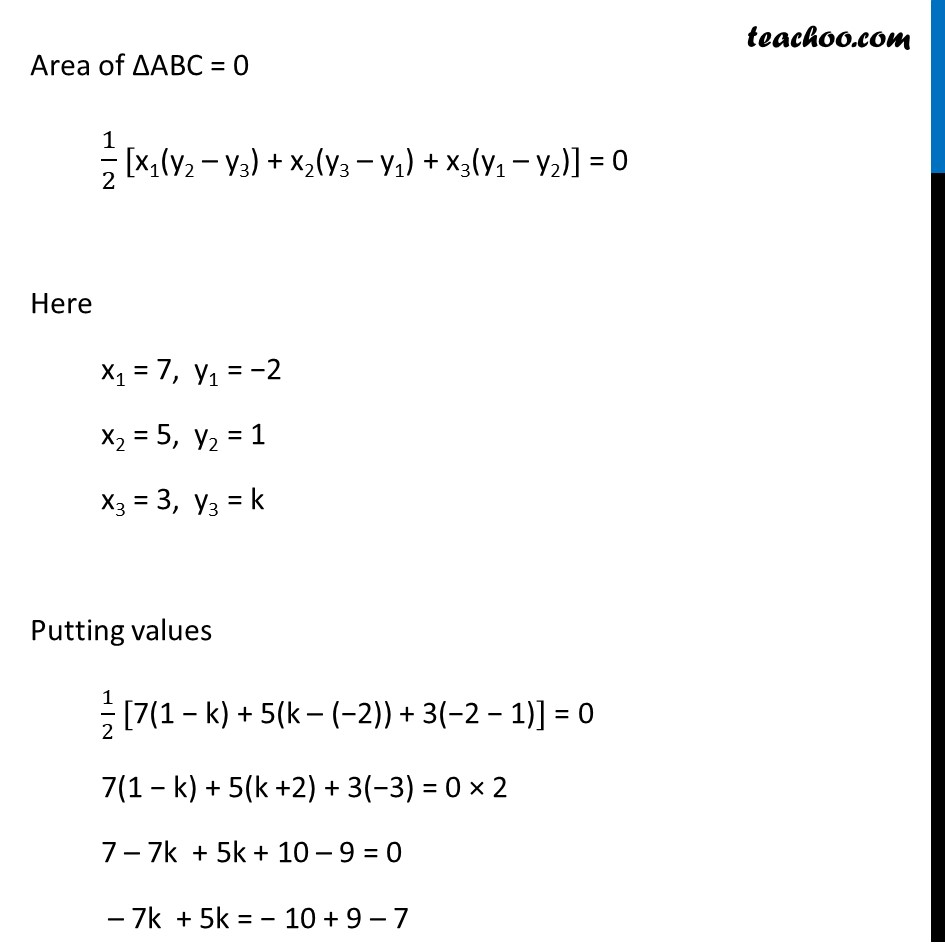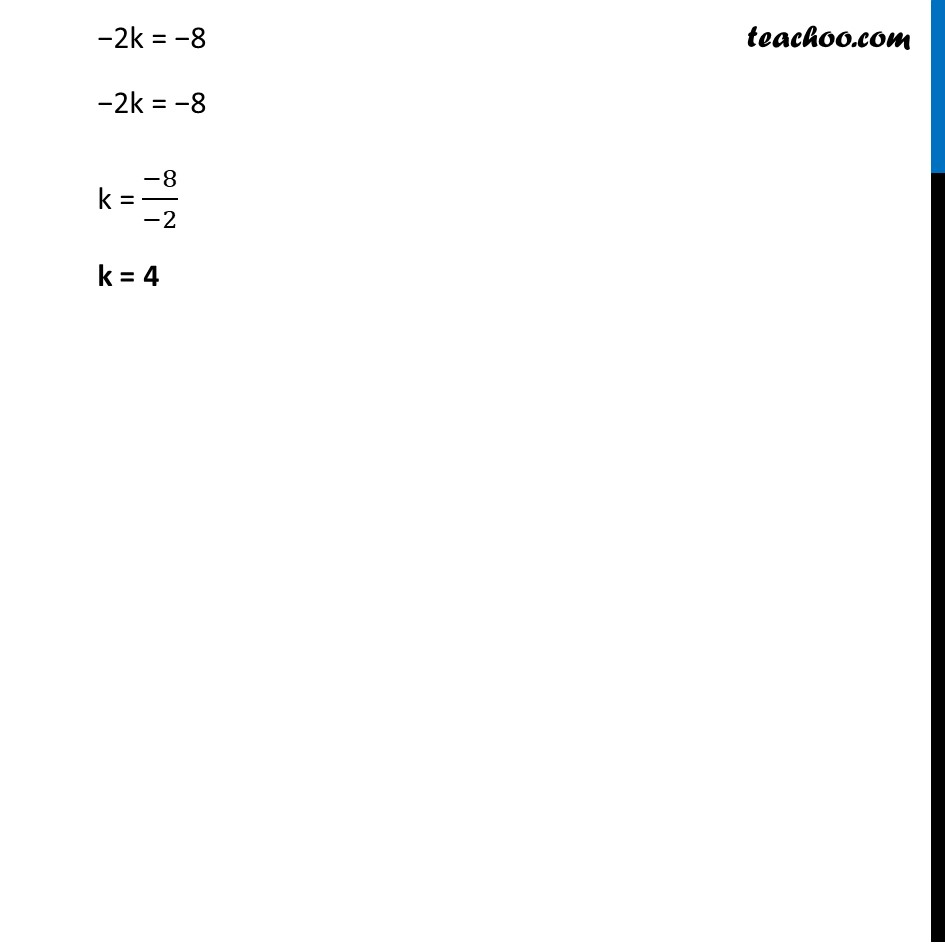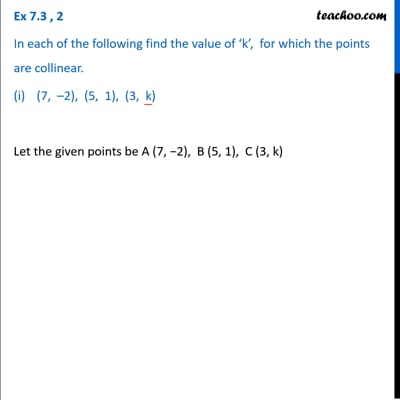Ex 7.3

Chapter 7 Class 10 Coordinate Geometry
Serial order wiseThis video is only available for Teachoo black users

### Transcript

Ex 7.3 , 2 In each of the following find the value of ‘k’, for which the points are collinear. (7, –2), (5, 1), (3, k) Let the given points be A (7, −2), B (5, 1), C (3, k) If the above points are collinear, they will lie on the same line, i.e. the will not form triangle or We can say that Area of ∆ABC = 0 1/2 [x1(y2 – y3) + x2(y3 – y1) + x3(y1 – y2)] = 0 Here x1 = 7, y1 = −2 x2 = 5, y2 = 1 x3 = 3, y3 = k Putting values 1/2 [7(1 − k) + 5(k – (−2)) + 3(−2 − 1)] = 0 7(1 − k) + 5(k +2) + 3(−3) = 0 × 2 7 – 7k + 5k + 10 – 9 = 0 – 7k + 5k = − 10 + 9 – 7 −2k = −8 −2k = −8 k = (−8)/(−2) k = 4